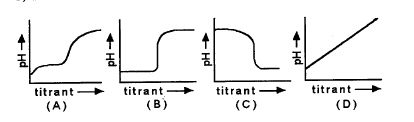Name:    Practice Test 3B

Multiple Choice

1.

What is the molar solubility of Cr(OH)3 in a saturated solution? (Ksp = 6.7 x 10–31)
 A) 1.7 x 10–8 M B) 1.3 x 10–8 M C) 3.9 x 10–8 M D) 8.2 x 10–16 M

2.

When a weak acid is titrated with a weak base, the pH at the equivalence point
 A) is greater than 7.0 B) is less than 7.0 C) is equal to 7.0 D) is determined by the size of Ka and Kb

3.

A 25.0-mL sample of 1.00 M NH3 is titrated with 0.15 M HCl.  What is the pH of the solution after addition of 15.0 mL of HCl? (Kb for NH3 = 1.8 x 10–5)
 A) 10.26 B) 9.21 C) 8.25 D) 9.3

4.

A change in pH affects the solubility of which of the following compounds?
 A) CuCl B) AgI C) BaF2 D) None of the above

5.

What volume of 0.500M H2SO4 must be added to 20.0 mL of 0.400M LiOH to reach the equivalence point?
 A) 8.00 mL B) 4.00 mL C) 16.0 mL D) 32.0 mL

6.

Which of the following statements is correct if 0.015 mol of KOH is added to 1.00L of 0.0010 M Ca(NO3)2? (Ksp for Ca(OH)2 = 6.5x10–6)
 A) Calcium hydroxide precipitates until solution is saturated. B) The solution is unsaturated and no precipitate forms. C) The concentration of calcium ions is reduced by the concentration of the hydroxide ions. D) Ksp for calcium nitrate must be known to make any meaningful predictions.

7.

Which solution will cause the greatest change in the solubility of PbCl2 at 25 °C?
 A) 0.1 M KNO3 B) 0.1 M CaCl2 C) 0.1 M NaCl D) 0.1 M HCl

8.

Which of the following diagrams best represents the titration curve for titration of a strong base by a strong acid?A) A B) B C) C D) D

9.

What is the solubility of PbI2 in 0.025M KI solution? (Ksp = 7.9 x10–9)
 A) 4.5 x10–2 M B) 1.3 x10–5 M C) 2.8 x10–2 M D) 8.9 x10–5 M

10.

Which of the following indicators would be the best to use when benzoic acid (Ka= 6.6 x 10–5) is titrated with NaOH?
 A) alizarin, pH range = 5.7 – 7.2 B) bromocresol green, pH range = 3.8 – 5.4 C) bromophenol blue, pH range = 3.0 – 4.5 D) phenol red, pH range = 6.9 – 8.2

11.

A solution is prepared by adding 0.10 mol of Fe(NO3)3 to 1 L of water.  Which of the following statements is true?
 A) The solution is basic B) The solution is acidic C) The solution is neutral D) No meaningful predictions can be made without knowing Ka and Kb values

12.

The solubility of aluminum hydroxide ___________ when dilute nitric acid is added to it.
 A) decreases B) increases C) does not change D) cannot be predicted based on information given

13.

Which of the following substances has the greatest solubility in water?
 A) MgCO3 , Ksp = 3.5 x 10–8 B) AgIO3 , Ksp = 3.1 x 10–8 C) NiCO3 , Ksp = 1.3 x 10–7 D) CuBr , Ksp = 5.0 x 10–9

14.

The concentration of the complex ion in each of the following solutions is 1.00 M.  In which of the solutions will the concentration of the metal ion be the greatest?
 A) CdI42– , Kf = 1.0 x 106 B) Cu(NH3)42+ , Kf = 5.6 x 1011 C) Zn(OH)42– , Kf = 3.0 x 1015 D) Be(OH)42– , Kf = 4.0 x 1018

15.

What is the maximum amount of sodium sulfate that can be added to 1.00 L of 0.0020 M Ca(NO3)2 before precipitation of calcium sulfate begins?  (Ksp = 2.4 x 10–5 for CaSO4)
 A) 4.8 x 10–8 mol B) 3.5 x 10–5 mol C) 4.9 x 10–3 mol D) 1.2 x 10–2 mol